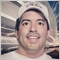# Explanation of the steps to calculate QQE Fast/Slow lines7

I am currently using the attached QQE indicator, successfully.  I have just gotten to a point where I want to understand the steps involved in it's calculation.  I have a reasonable grasp of how to code an indicator, but this one seems a bit more complex than what I am use to.  In particular, this block of code is where I am getting stuck:

```   double Stop1=QQE[limit];
double Stop2=QQE[limit];

for(i=limit; i>=0; i--){
if(QQE[i] < TS1[i+1])
{
Stop1=QQE[i] + DELTA[i]*Fast_ATR_Multipliers;
if (Stop1 > TS1[i+1])
{
if ( QQE[i+1] <  TS1[i+1])
{
Stop1 = TS1[i+1];
}
}
}

else if  ( QQE[i] > TS1[i+1] )
{
Stop1=QQE[i] - DELTA[i]*Fast_ATR_Multipliers;
if (Stop1 < TS1[i+1] )
{
if (QQE[i+1] >  TS1[i+1])
{
Stop1 = TS1[i+1];
}
}

}
TS1[i]=Stop1;
}```

If it's not beyond the scope of this forum, I'd like a walk through of what is going on in the above.  I have read a few explanations of how the QQE is calculated, but they seem to gloss over how the trailing lines are calculated.

Files:371

gershgorin:

I am currently using the attached QQE indicator, successfully.  I have just gotten to a point where I want to understand the steps involved in it's calculation.  I have a reasonable grasp of how to code an indicator, but this one seems a bit more complex than what I am use to.  In particular, this block of code is where I am getting stuck:

If it's not beyond the scope of this forum, I'd like a walk through of what is going on in the above.  I have read a few explanations of how the QQE is calculated, but they seem to gloss over how the trailing lines are calculated.

I never have looked or studied anything about QQE, but I can understand the above code at this first look..

I will explain what it does, and I hope it make some sense to you since you know the QQE theory.. :)

The code above, gets the QQE level and compare it to the TSI level of the Candle next to it, and permits the QQE level oscilate based on a variant delta,

but it locks the maximum and minimum levels, up to the TSI levels of the last candle.

In other words, it is limiting the maximum and minimum ranges for QQE variation, like a Ceil and Floor based on the last candle TSI value.

It looks similar to as if we were compressing it somehow. between two edge limits, based on the value of the last candle.